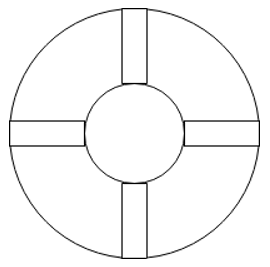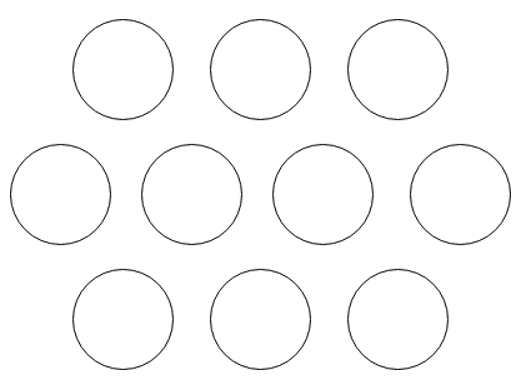Q.1
• a) True
• b) False
Q.2
• a) 1
• b) 2
• c) 3
• d) 4
Q.3
##### Which of the following has the maximum Log mean temperature difference for a Shell and tube Heat Exchanger?
• a) Counter-flow
• b) Parallel Flow
• c) Cross Flow
• d) Split Flow
Q.4
##### What are the types of baffles exist for a shell and tube heat exchanger?
• a) Cross baffle, Split baffle and segmental baffle
• b) Counter baffle, doughnut baffle and Cross baffle
• c) Orifice baffle, segmental baffle and doughnut baffle
• d) Orifice baffle, segmental baffle and Counter baffle
Q.5
• a) Segmental
• b) Orifice
• c) Doughnut
• d) Divided
Q.6
##### What are the types of pitch arrangements are available for the baffles to be used in Shell and Tube HE?
• a) Square pitch and Rectangular pitch
• b) Square pitch and Triangular pitch
• c) Rectangular pitch and Hexagonal pitch
• d) Hexagonal pitch & Triangular pitch
Q.7
• a) True
• b) False
Q.8
##### Which one of the following fluid cannot be placed in the shell side?
• a) Condensing Vapour
• b) Fluid with very high temperature
• c) Fluid with very high pressure
• d) Fluid with high viscosity
Q.9
##### Which one of the following fluid cannot be placed in the tube side?
• a) Fouling Fluid
• b) Cooling liquid
• c) Corrosive fluid
• d) Highly viscous
Q.10
##### When the fluid is very viscous, we can __________
• a) Keep it in shell side
• b) Keep it in tube side
• c) Keep it in any side
• d) Not use it
Q.11
##### To calculate the temperature difference in a Shell and tube heat exchanger, we use _____
• a) LMTD
• b) Mean temperature difference
• c) Median of the temperature difference
• d) Square mean of the temperature difference
Q.12
##### Recognize the type of pitch given the figure below.
• a) Square pitch
• b) Triangular pitch
• c) Rotated Square pitch
• d) Hexagonal pitch
Q.13
##### Recognize the type of pitch given the figure below.• a) Square pitch
• b) Triangular pitch
• c) Rotated Square pitch
• d) Hexagonal pitch
Q.14
##### Recognize the type of pitch given the figure below.• a) Square pitch
• b) Triangular pitch
• c) Rotated Square pitch
• d) Hexagonal pitch